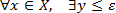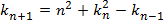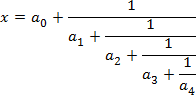﻿ TRVMathItemInfo.Text

# TRVMathItemInfo.Text

Mathematical expression in LaTeX-like format.

property Text: TRVUnicodeString;

Differences from LaTeX:

the symbols "[]{}" after "\left" and "\right" must be prefixed with "\"  (i.e. "\left\[" instead of "\left[" in LaTeX);

matrices are defined as "\matrix{ a_11 & a_12 \\ a_21 & a_22}"

conditions are defined as "\case{ a_11 & a_12 \\ a_21 & a_22}"

Unlike text items, this is not the text returned by TCustomRichView.GetItemText.

Use TRichViewEdit.SetCurrentItemExtraStrPropertyEx to change value of this property as an editing operation (RVMathItem.rvespcText).

Examples of values and results:

\forall x \in X, \quad \exists y \leq \epsilonk_{n+1} = n^2 + k_n^2 - k_{n-1}x = a_0 + \frac{1}{\displaystyle a_1 + \frac{1}{\displaystyle a_2 + \frac{1}{\displaystyle a_3 + \frac{1}{a_4} } } }If the expression is incorrect, the item is displayed in an erroneous state.